# YruRU Method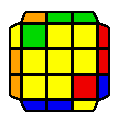##### R' U2 R U2 R U R' U' R' U R U2 R U2 R'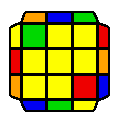##### (U) R U R' U R U' R' U R U' R' U R U2 R'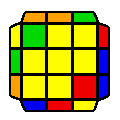##### (U') R U R' U R U2 R' U2 R U2 R' U' R U' R'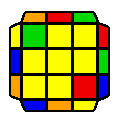##### (U') R U2 R' U' R U' R' U2 R U R' U R U2 R'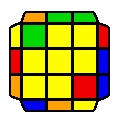##### R2 U' R U' R U2 R U R U' R2 U' R' U R2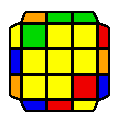##### R2 U' R U' R U R' U R U R' U' R' U R2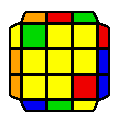##### R' U R' U R2 U' R2 U' R2 U' R U R' U' R'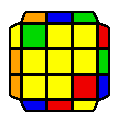##### R' U2 R' U' R2 U' R U R' U' R U2 R U' R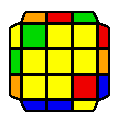##### (U) R U2 R U R2 U R' U' R U R' U2 R' U R'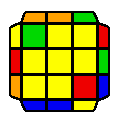##### (U') R U R' U R U2 R' U R' U' R U' R' U2 R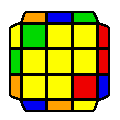##### R' U R' U2 R' U R U' R' U R2 U R U2 R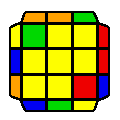##### (U') R U2 R' U' R U' R' U R' U2 R U R' U R

Average movecount: 15.0

Average double turns: 2.7

Algorithms: 12

All L cases are 15 moves long.

If a large 2x2 block is solved, it is the 2-twist case. If there are no blocks (2 cases) then the 2x2 blocks will look like either an H perm or a Z perm.

If there are two blocks; then they may look like a Y perm (1 case) which is simply two sune algorithms connected with a U move; or a J perm (2 cases), i.e. either a Ja or a Jb; or a T perm (2 cases) i.e. either a vertical T perm or a horizontal T perm. The horizontal T perm and the Jb perm are simply 2 sune algorithms connected with a U move.

The 1 block cases (4 cases) can be identified by the position of the block.

Note, these rules are only to make learning of these cases simpler. For speed, a better recognition system is to be adopted.